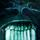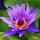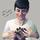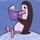## Social Question# Can anyone explain what NP-hard means?

Asked by Lightlyseared (32145) April 13th, 2016

So I’ve just discovered that something I do every month is known to have NP-hard complexity (Nurse scheduling). Any one care to have a go explaining what that means? And preferably do a better job than Wikipedia page.
I’m guessing (from experience) that it means there’s no simple way of getting to a correct answer but that once you have an answer it’s easy to tell if it’s correct (by the number of angry people trying to kill me)...

Observing members: 0Composing members: 0NP-hardness (non-deterministic polynomial-time hard), in computational complexity theory, is a class of problems that are, informally, “at least as hard as the hardest problems in NP”. More precisely, a problem H is NP-hard when every problem L in NP can be reduced in polynomial time to H.[clarification needed]:80 As a consequence, finding a polynomial algorithm to solve any NP-hard problem would give polynomial algorithms for all the problems in NP, which is unlikely as many of them are considered hard.

A common mistake is thinking that the NP in “NP-hard” stands for “non-polynomial”. Although it is widely suspected that there are no polynomial-time algorithms for NP-hard problems, this has never been proven. Moreover, the class NP also contains all problems which can be solved in polynomial time.

Tropical_Willie (26827)“Great Answer” (1) Flag as…You said, “I’m guessing (from experience) that it means there’s no simple way of getting to a correct answer but that once you have an answer it’s easy to tell if it’s correct.” That’s the definition of an NP problem – the problem cannot necessarily be solved efficiently (by efficiently we mean in at least polynomial time), but given a proposed answer, we can check whether it is correct efficiently.

NP-hard is the set of all problems that are at least as hard as NP problems. So those that cannot necessarily be solved efficiently, but can be checked efficiently, and those that cannot even necessarily be checked efficiently.

Mariah (25863)“Great Answer” (3) Flag as…@Tropical_Willie I read that. It didn’t help.

@Mariah care to explain polynomial time? ;)

Lightlyseared (32145)“Great Answer” (0) Flag as…Sure! Time complexity refers to the amount of time it takes your algorithm to run in comparison to the size of the data it’s operating on.

Say you’re developing an algorithm where the input is a list of length n. If your solution involves iterating through the list once, it would be said to be in linear time (O(n)) because the speed of the algorithm will increase linearly as the size of n increases. If your solution involves comparing each item of the list with each other item in the list, the time complexity is O(n^2) because as n increases, the computation time of the algorithm increases proportional to the square of n. Polynomial time would be a solution in O([any arbitrary polynomial]).

Mariah (25863)“Great Answer” (4) Flag as…I taught her everything I know.

janbb (57228)“Great Answer” (1) Flag as…